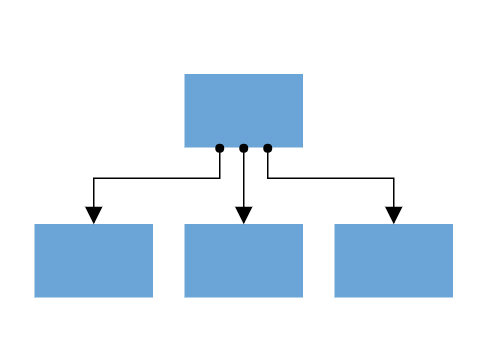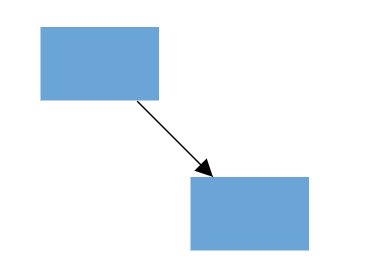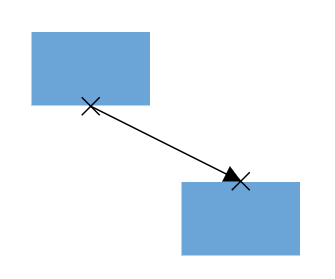# Ports

4 Apr 202223 minutes to read

Diagram provides support to define custom ports for making connections.When a connector is connected between two nodes, its end points are automatically docked to the node’s nearest boundary.Ports act as the connection points of the node and allows to create connections with only those specific points.## Add ports when initializing nodes

To add a connection port, define the port object and add it to node’s ports collection. The `offset` property of port accepts an object of fractions and used to determine the position of ports.

``````using System;
using System.Collections.Generic;
using System.Linq;
using System.Web;
using System.Web.Mvc;
using Syncfusion.EJ2.Diagrams;
using System.Drawing;

namespace EJ2MVCSampleBrowser.Controllers.Diagram {
public partial class DiagramController: Controller {
// GET: Nodes
public ActionResult Nodes() {

List < DiagramPort > ports1 = new List < DiagramPort > ();
Id = "port1", Shape = PortShapes.Circle,
Offset = new DiagramPoint() {
X = 0.5, Y = 0.5
}
});
List < DiagramNode > nodes = new List < DiagramNode > ();
Id = "node1",
Width = 100,
Height = 100,
Style = new NodeStyleNodes() {
Fill = "#6BA5D7",
StrokeColor = "White"
},
text = "node1",
OffsetX = 100,
OffsetY = 100,
Ports = ports1
});
ViewBag.nodes = nodes;

return View();
}
}
public class Node: DiagramNode {
public string text;
}
}``````

Add ports at runtime by using the client-side method `addPorts`.

The port’s ID property is used to define the unique ID for the port and its further used to find the port at runtime. If ID is not set, then default ID is automatically set.

``````using System;
using System.Collections.Generic;
using System.Linq;
using System.Web;
using System.Web.Mvc;
using Syncfusion.EJ2.Diagrams;
using System.Drawing;

namespace EJ2MVCSampleBrowser.Controllers.Diagram {
public partial class DiagramController: Controller {
// GET: Nodes
public ActionResult Nodes() {
List < DiagramNode > nodes = new List < DiagramNode > ();
Id = "node1",
Width = 100,
Height = 100,
Style = new NodeStyleNodes() {
Fill = "#6BA5D7",
StrokeColor = "White"
},
text = "node1",
OffsetX = 100,
OffsetY = 100,
});
ViewBag.nodes = nodes;

return View();
}
}
public class Node: DiagramNode {
public string text;
}
}``````
``````// Initialize port collection
var port= [{
id: 'port1',
offset: {
x: 0,
y: 0.5
},
visibility: PortVisibility.Visible
} {
id: 'port2',
offset: {
x: 1,
y: 0.5
},
visibility: PortVisibility.Visible
},
{
id: 'port3',
offset: {
x: 0.5,
y: 0
},
visibility: PortVisibility.Visible
},
{
id: 'port4',
offset: {
x: 0.5,
y: 1
},
visibility: PortVisibility.Visible
}
];

// Method to add ports through run time

## Remove ports at runtime

Remove ports at runtime by using client-side method `removePorts`.

``````using System;
using System.Collections.Generic;
using System.Linq;
using System.Web;
using System.Web.Mvc;
using Syncfusion.EJ2.Diagrams;
using System.Drawing;

namespace EJ2MVCSampleBrowser.Controllers.Diagram {
public partial class DiagramController: Controller {
// GET: Nodes
public ActionResult Nodes() {
List < DiagramPort > ports1 = new List < DiagramPort > ();
Id = "port1", Shape = PortShapes.Circle, Offset = new DiagramPoint() {
X = 0, Y = 0.5
}, Visibility = PortVisibility.Visible
});
Id = "port2", Shape = PortShapes.Circle, Offset = new DiagramPoint() {
X = 1, Y = 0.5
}, Visibility = PortVisibility.Visible
});
Id = "port3", Shape = PortShapes.Circle, Offset = new DiagramPoint() {
X = 0.5, Y = 1
}, Visibility = PortVisibility.Visible
});
Id = "port4", Shape = PortShapes.Circle, Offset = new DiagramPoint() {
X = 1, Y = 1
}, Visibility = PortVisibility.Visible
});

List < DiagramNode > nodes = new List < DiagramNode > ();
Id = "node1",
Width = 100,
Height = 100,
Style = new NodeStyleNodes() {
Fill = "#6BA5D7",
StrokeColor = "White"
},
text = "node1",
OffsetX = 100,
OffsetY = 100,
Ports = ports1
});
ViewBag.nodes = nodes;

return View();
}
}
public class Node: DiagramNode {
public string text;
}
}``````
``````var ports = [{
id: 'port1',
}, {
id: 'port2',
}, {
id: 'port3',
}, {
id: 'port4',
}]
diagram.removePorts(diagram.nodes, ports);``````

## Update port at runtime

You can change any port properties at runtime and update it through the client-side method `dataBind`.

``````using System;
using System.Collections.Generic;
using System.Linq;
using System.Web;
using System.Web.Mvc;
using Syncfusion.EJ2.Diagrams;
using System.Drawing;

namespace EJ2MVCSampleBrowser.Controllers.Diagram {
public partial class DiagramController: Controller {
// GET: Nodes
public ActionResult Nodes() {

List < DiagramPort > ports1 = new List < DiagramPort > ();
Id = "port1", Shape = PortShapes.Circle,
Offset = new DiagramPoint() {
X = 0.5, Y = 0.5
}
});
List < DiagramNode > nodes = new List < DiagramNode > ();
Id = "node1",
Width = 100,
Height = 100,
Style = new NodeStyleNodes() {
Fill = "#6BA5D7",
StrokeColor = "White"
},
text = "node1",
OffsetX = 100,
OffsetY = 100,
Ports = ports1
});
ViewBag.nodes = nodes;

return View();
}
}
public class Node: DiagramNode {
public string text;
}
}``````
``````diagram.nodes.ports.offset = {
x: 1,
y: 1
};
diagram.dataBind();``````

## Appearance

``````using System;
using System.Collections.Generic;
using System.Linq;
using System.Web;
using System.Web.Mvc;
using Syncfusion.EJ2.Diagrams;
using System.Drawing;

namespace EJ2MVCSampleBrowser.Controllers.Diagram {
public partial class DiagramController: Controller {
// GET: Nodes
public ActionResult Nodes() {

List < DiagramPort > ports1 = new List < DiagramPort > ();
Id = "port1", Shape = PortShapes.Circle,
Offset = new DiagramPoint() {
X = 0.5, Y = 0.5
},
style = new DiagramShapeStyle() {
Fill = "Black", StrokeColor = "Red", StrokeWidth = 2
},
Visibility = PortVisibility.Visible
});
List < DiagramNode > nodes = new List < DiagramNode > ();
Id = "node1",
Width = 100,
Height = 100,
Style = new NodeStyleNodes() {
Fill = "#6BA5D7",
StrokeColor = "White"
},
text = "node1",
OffsetX = 100,
OffsetY = 100,
Ports = ports1
});
ViewBag.nodes = nodes;

return View();
}
}
public class Node: DiagramNode {
public string text;
}
}``````

## Offset

The offset property of port is used to align the port based on fractions. 0 represents top/left corner, 1 represents bottom/right corner, and 0.5 represents half of width/height.

## Constraints

The constraints property allows to enable/disable certain behaviors of ports. For more information about port constraints, refer to `Port Constraints`.